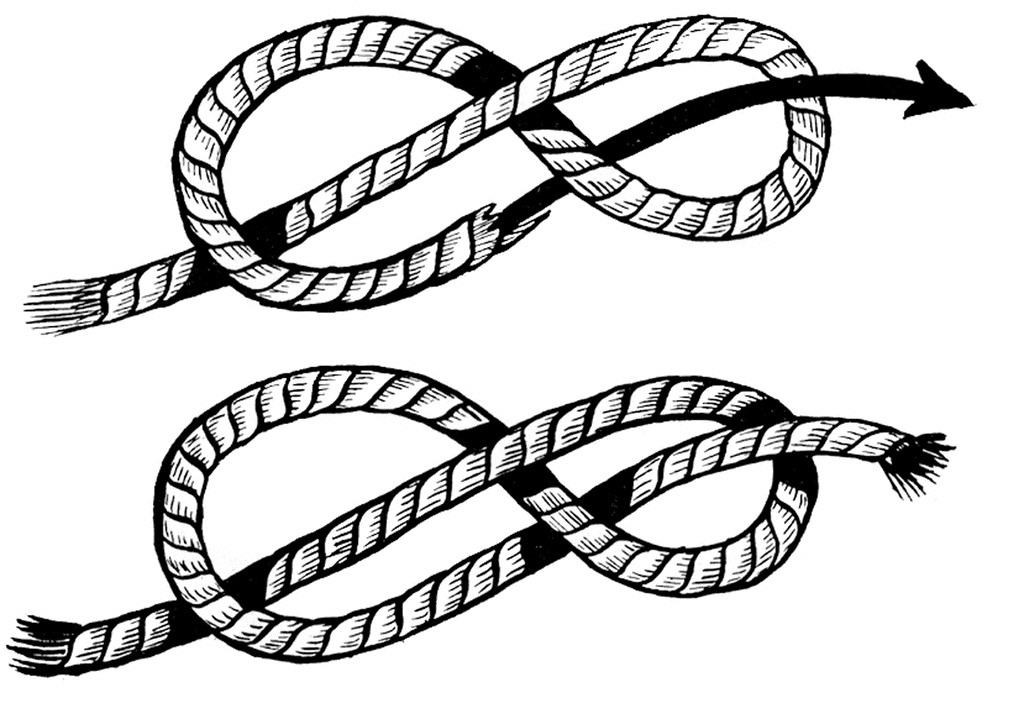# How to see isometries of figure 8 knot complement

The figure 8 knot complement $M$ is the orientable double cover of the Gieseking manifold, which implies that $M$ has a fixed-point free involution. If we think of $M$ with its hyperbolic metric, this involution is an isometry. Is there some way of visualizing this isometry?

I know how to produce one relatively easy to see isometry of $M$. The fundamental group of $M$ is a two generator group. Lift geodesic representatives of a pair of generators to $\mathbb{H}^3$. These geodesics have a mutual perpendicular, and $180^{\circ}$ rotation about that geodesic descends to an involution of $M$. However, this map has fixed points, and I'd like to "see" one that doesn't.

If you're interested in the involution only defined on the complement, Igor's answer does a fine job.

But the involution extends to an involution of $S^3$ and perhaps you'd like to see that?

I think the symmetry is a little tricky to traditionally visualize, because as a map of $S^3$, thought of as the unit sphere $S^3 \subset \mathbb C^2$ it's of the form $(z_1,z_2) \longmapsto (\overline{z_1}, -z_2)$. If you stereographically project at one of these fixed points, this becomes the involution of $\mathbb R^3$ given by $(x,y,z) \longmapsto (-x,-y,-z)$. Both the fixed points have to be on the knot, so this means we have to find a "long" embedding of the figure-8 knot in $\mathbb R^3$ which is invariant under the antipodal map. That's easy. Here are two such (approximate) positions:In the latter picture the fixed point is easier to see -- the blue straight line intersects the knot in 5 points, one at the "center" and four other points indicated by blue balls.

Looking through the code I used to generate the latter picture, the parametrization I use of the figure-8 knot is:

$$t \longmapsto (5(t^3-3t), 0.25(t^7-42t), t^5-5t^3+4t)$$

which is easy to verify is equivariant with respect to the above involution, as all the terms in the polynomial are odd.

• Nice! What did you use to generate the picture? Nov 19, 2011 at 0:45
• The latter image was generated in two steps. The knot and the line were computed in some C++ code that I wrote. Then the 3-dimensional data was output into a PoVRay format, which was then rendered in PoVray: povray.org The top image I just grabbed in a Google search. Nov 19, 2011 at 1:49
• Maybe I'm confused; isn't the OP trying to visualize a fixed-point free involution?
– j.c.
Nov 19, 2011 at 6:14
• The only fixed points are on the knot -- it's free on the knot complement. Nov 19, 2011 at 6:24

The figure 8 complement decomposes into 2 regular ideal tetrahedra (see Thurston's notes, here for example.) This gives the involution quite explicitely.

• Small comment: one can arrange that the gluing maps from the 1st tetrahedron to the 2nd are the ones that send the triangle opposite vertex $i$ to the triangle opposite vertex $i+2 \ mod \ 4$. Moreover, on those triangles the gluing map is the one that preserves the order of the vertices (coming from their labelling in the ambient tetrahedron with vertices $\{0,1,2,3\}$). So if you switch the two tetrahedra around (identity on vertices), it descends to an involution of the underlying manifold. One still has to check that there's no fixed points on the interior of the edges or triangles. Jan 26, 2015 at 16:19

In terms of 'seeing' the isometry $\rho$ needed to realize the Gieseking manifold as a quotient of the figure 8 knot complement $S^3-K$, we require a slight of hand, namely thinking of another name for the manifold. The reason for this is simple, the Gieseking manifold is non-orientable so $\rho$ must reverse orientation. However, there are no non-orientable manifolds covered by $S^3$, so we will not be able to 'see' $\rho$ acting on $S^3$.

Instead, we can think of $S^3-K$ by another name as follows. The figure 8 knot complement is fibered. In fact, it can be thought of as a once-punctured torus bundle with monodromy given by $\pmatrix{2 & 1\\ 1 & 1}$. It turns out $\rho$ acts on once-punctured torus bundle by a glide reflection (loosely translate 'half-way' up and reflect).

• Thanks Neil I was looking for this kind of approach. In terms of the generators of the boundary then, the longitude L is sent to -L, and M to -M? i.e. do you also flip the "vertical" circle direction in the bundle?
– Tali
Aug 18, 2021 at 4:34
• @Tali - The "glide reflection" sends $L$ to $-L$ and sends $M$ to $M$. (Here I am using $L$ to denote the boundary of the Seifert surface and $M$ to denote the meridian of the knot.) You can see this by realising the matrix $\begin{pmatrix}2 &1\\ 1 & 1 \end{pmatrix}$ as the square of $\begin{pmatrix}1 &1\\ 1 & 0 \end{pmatrix}$. Jul 12, 2022 at 17:57